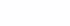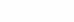# Optuna. Selection of hyperparameters for your model

Hyperparameters are characteristics of the model that are fixed before the start of training (for example, the depth of the decision tree, the value of the regularization strength in a linear model, the learning rate for gradient descent). Hyperparameters, in contrast to the parameters, are set by the model developer before training it, in turn, the model parameters are adjusted in the process of training the model on the data.

Optuna is a framework for automated search for optimal hyperparameters for machine learning models. She selects these parameters by trial and error.

Key features of the framework:

1. Custom hyperparameter search space. The developer can independently set the space for searching for hyperparameters using the basic Python syntax (loops, conditions).

2. SoTA algorithms for choosing hyperparameters from a space specified by the developer (samplers) and for early termination of unpromising experiments (pruners). Optuna provides various sampling and pruning algorithms, the developer can choose a specific one, leave the default one, or write his own.

3. Ease of parallelization of the process of searching for hyperparameters. You can also attach a dashboard with real-time learning visualization to Optuna.

## Installation

Recommended installation via pip.

``pip install optuna``

## Basic Example

This framework is usually used as a hyperparameter optimizer, but no one forbids using it to optimize any function. As a basic use case, the authors of the framework show how a quadratic function can be minimized. `.`

``````import optuna

def objective(trial):
x = trial.suggest_float('x', -10, 10)
return (x - 2) ** 2

study = optuna.create_study()
study.optimize(objective, n_trials=100)

study.best_params  # E.g. {'x': 2.002108042}``````
1. Define the objective function `objective` in through arguments it will receive a special object `trial`. With it, you can assign various hyperparameters. For example, as in the example above, we set `x` in the interval.

2. Next, we create a learning object using the method `optuna.create_study`.

3. We start the optimization of the objective function `objective` for 100 iterations `n_trials=100`. There are 100 calls to our function with various parameters from -10 to 10. Which parameters optuna chooses will be described below.

## How to define the hyperparameter search space?

As shown above, a special object will be passed to the target function `Trial`. Since our target function will be called a certain number of times, on each call from the object `Trial` new parameter values ​​will be returned. The developer can only set the characteristics of these parameters. There are several methods for this:

1. `suggest_categorical(name, choice) `specifies categorical parameters. Example

2. `suggest_float(name, low, high, *, step=None, log=False)` sets the type parameter `float` – floating point number. Example

3. `suggest_int(name, low, high, step=1, log=False)` sets the type parameter `int` is an integer. Example

## What else can be configured before optimization?

To start training, we need to create an object `Study.` It is recommended to create it either using the method `create_study` (example) or `load_study` (example).

At the time of creation, you can specify:

1. function optimization direction `directions`– minimization or maximization

2. `storage` database address for saving test results

3. `study_name` name, if not specified, it will be generated automatically. Specifying your own name, convenient when saving experiments and loading them

4. `pruner` and `sampler` – about it below

After creating the Study object, you can start optimizing the objective function. This can be done using the method `optimize` (example).

### How to view optimization results?

The Study object has special fields that allow you to view the results after training:

1. `study.best_params` best options

2. `study.best_value` best optimal objective function value

3. `study.best_trial` expanded parameters of the best test

## How to save/load test results?

Save only the history as a dataframe

``````df = study.trials_dataframe()
df.to_csv('study.csv')

Save a dump of the optimizer itself

``````joblib.dump(study, 'experiments.pkl')

You can also save test results in the database, for this Optuna has a special module Storages, which provides some objects for DB interaction. For example, there is an object that allows you to interact with redis. Example.

## What is Sampler and Pruner?

samplers in Optuna, it is a set of algorithms for finding hyperparameters.

A small digression into the theory. There are various approaches to finding optimal hyperparameters, below are examples of algorithms:

1. Grid Search – grid search. For each hyperparameter, a list of possible values ​​is specified, after which all possible combinations of elements of the lists are sorted out, the set on which the value of the objective function was minimum/maximum is selected.

2. random search – random search. For each hyperparameter, a distribution is specified from which its value is selected. Thanks to this approach, it is possible to find the optimal set of hyperparameters faster.

3. Bayeian optimization. An iterative method that, at each iteration, indicates the most likely point at which our objective function will be optimal. In this case, the output probable points include two components:

1. a good point where, according to history, the function produced good results on previous calls (exploitation)

2. a good point where there is high uncertainty, that is, unexplored parts of space (exploration)

More details about these algorithms, as well as about the Tree-structured Parzen Estimator (TPE), Population Based Training (PBT) can be found in textbook on machine learning from Yandexthere you can also find links to useful resources on this topic and a comparison of approaches with each other.

Optuna implements:

The default is set TPESampler.

pruners in Optuna, it is a set of algorithms for thinning out experiments. Pruning is a mechanism that allows you to abort experiments that are highly likely to lead to suboptimal results.

For example, consider the simplest pruner – MedianPruner. He cuts off at every step half of the unpromising trials.At each epoch (step) Pruner discards exactly half of the tests, after 3 epochs, the 7th test remains the best, it will be completed, the rest will be completed earlier.

Optuna implements:

• MedianPruner – pruner using the rule half stops, half continues

• NopPruner – a pruner who never stops testing.

• PatientPruner – pruner wrapper over any other pruner, allows you not to stop unpromising tests, until PatientPruner runs out of patience a few more epochs.

• PercentilePruner – pruner that saves a certain percentile of trials.

• HyperbandPruner – algorithm Hyperband

• ThresholdPruner – pruner, which stops the test if the value of the objective function is out of bounds – it exceeded the upper threshold or became lower than the lower threshold.

## Which Sampler and Pruner should I use?

In the documentation according to this study Benchmarks with Kurobako For non-deep learning, you should use:

The documentation also provides recommendations for deep learning.

## How to make friends with popular libraries?

Optuna has module `integration`, which contains classes used to integrate with external popular machine learning libraries. Among them are such libraries as CatBoost, fast.ai, Keras, LightGBM, PyTorch, scikit-learn, XGBoost. The full list can be found here.

## What else is there?

• There is a module for visualization, it provides functions for plotting the optimization process using plotly and matplotlib. Plotting functions usually take a Study object and settings.

Here an example of plotting the optimization history.

• There is a module importancewith the help of it it is possible to evaluate the importance of hyperparameters based on completed tests.